Journal DetailsFormat
Journal
eISSN
2083-134X
First Published
16 Apr 2011
Publication timeframe
4 times per year
Languages
EnglishOpen Access

# Kinetics of oxygen adsorption on ZnS nanoparticles synthesized by precipitation process

###### Accepted: 19 Jan 2016
Journal DetailsFormat
Journal
eISSN
2083-134X
First Published
16 Apr 2011
Publication timeframe
4 times per year
Languages
English

ZnS nanoparticles were synthesized through a one-step precipitation process. Effect of time and temperature on the formation reaction was investigated. The synthesized samples were characterized by X-ray diffraction (XRD), ultraviolet (UV) visible absorption and photoluminescence (PL) spectrophotometry. Based on XRD and UV-Vis data, the particles produced at 70 °C had a mean particle size of about 5 nm. Increasing time and temperature of the synthesis reaction resulted in photoluminescence intensification. PL spectroscopy helped understanding the adsorption kinetics of oxygen on ZnS nanoparticles during the precipitation synthesis process. Fabrication of ZnS structures with appropriate oxygen adsorption capacity was suggested as a means of PL emission intensity control.

#### Key words

Introduction

ZnS nanoparticles have many important applications, such as in-vivo and in-vitro imaging of target tissues . Their fluorescence properties have been vastly studied . Based on the specific application, appropriate morphology and particle sizes have been obtained by tuning precursor concentration, stabilizing surfactant, pH and temperature of the chemical processes generally used for production of the ZnS particles . Recently, dual florescence-MR cellular and molecular imaging has been investigated using ZnS:Mn nanoparticles [18, 19]. Addition of manganese to ZnS as a doping element leads to a red shift in the emission peak of the PL spectrum [20, 21]. On the other hand, reduction of the ZnS particle size leads to the blue shift of the PL emission peak with respect to the bulk ZnS . In many studies, an enhancement in the luminescence intensity has been reported with the reaction time . The luminescence intensity is strongly affected by variations in the surface states such as oxygen adsorption and particles crystallinity .

So far, the doped ZnS nanoparticles, like Mn doped ZnS quantum dots and ZnS containing core/shell nanostructures such as CdSe/ZnS, InP/ZnS and CdTe/CdS/ZnS nanoparticles have been widely used for cell imaging [23, 24]. The red shift of the PL spectra of these nanostructures with respect to that of ZnS quantum dots makes them more suitable for in-vitro and in-vivo imaging applications; especially due to increasing of the beam penetration depth. Their emission intensity is affected by their surface defects.

The main goal of the present work was the synthesis of ZnS nanoparticles with suitable photoluminescence properties via a simple chemical route. This purpose has been satisfied and verified via TEM results. Another important aim was to investigate the kinetics of variation of the surface defects. This one is very important for controlling and predicting surface oxygen content. ZnS nanoparticles were therefore synthesized by a one-step precipitation method carried out at two temperatures: 70 °C and 90 °C. The PL spectra of the synthesized particles were determined at different reaction durations from 30 to 240 minutes. Using UV and XRD patterns, the particle sizes were evaluated. Kinetics of oxygen adsorption was studied by analyzing of the PL spectra at the above mentioned temperatures. Increasing the PL intensity at a constant wavelength was taken as an indication of the increase of the surface oxygen. The results indicate that the Avrami kinetic model governs the oxygen adsorption process.

Experimental
Materials and methods

All chemicals were of analytical grade and were used in the as-received condition without further purification. Water soluble ZnS quantum dots were synthesized in an aqueous medium under atmospheric air by adding 0.320 mL of 3-mercaptopropionic acid (MPA) to 0.1 M Zn(CH3COO)2·2H2O (20 mL) which was used as the capping agent. The pH of the solution was raised up to 5.5 by addition of 2M NaOH. An oil bath was used to heat up the (Zn2+/MPA) solution to the reaction temperature (70 °C and 90 °C). An aqueous solution of 1 M Na2S·9H2O (20 mL) having the same temperature as the oil bath was then injected into the reaction flask and the mixture was refluxed. Sampling was done at different times (t = 30 to 240 minutes) with subsequent cooling to the room temperature. The obtained samples were characterized by XRD, UV-Vis absorption and PL spectroscopy. The experimental conditions of preparing the twelve samples used in this study are summarized in Table 1.

Experimental conditions of synthesizing samples A1 to A6 and B1 to B6.

t [min] →306090120150180210240
T [°C] ↓
70A1A2A3(S)A4A5A6
90B1B2B3B4B5B6
Characterization

Structure and florescence properties of the synthesized ZnS particles were investigated by XRD (Siemens D5000 X-ray theta/theta diffractometer) using high-intensity CuKα radiation via the movement of both the X-ray source and the detector. The XRD scan rate was fixed at 0.02° 2θ/s with a step size of 0.02°. The particle size was calculated through the Scherrer equation employing the highest intensity peak. The UV-Vis absorption recording and the PL intensity measurements were performed at 25 °C using the Varian Cary 100 UV-Vis and Eidingberg spectrophotometers, respectively. A JEOL TEM JEM-2010F was used to determine the average particle size and morphology of the particles at an accelerating voltage of 150 kV.

Results and discussion

The XRD pattern of the sample S synthesized at 70 °C for 90 minutes is shown in Fig. 1a. The characteristic peaks located at 28.48°, 48.07° and 56.17° are related to (1 1 1), (2 2 0) and (3 1 1) planes in the ZnS lattice, respectively. All the XRD peaks confirm the cubic zinc blende structure of ZnS nanoparticles. Considering the highest intensity peak located at 24.48°, the mean particles size was estimated by Scherrer equation:

$d=kλβcosθ$$$d = {{k\lambda } \over {\beta \cos \theta }}$$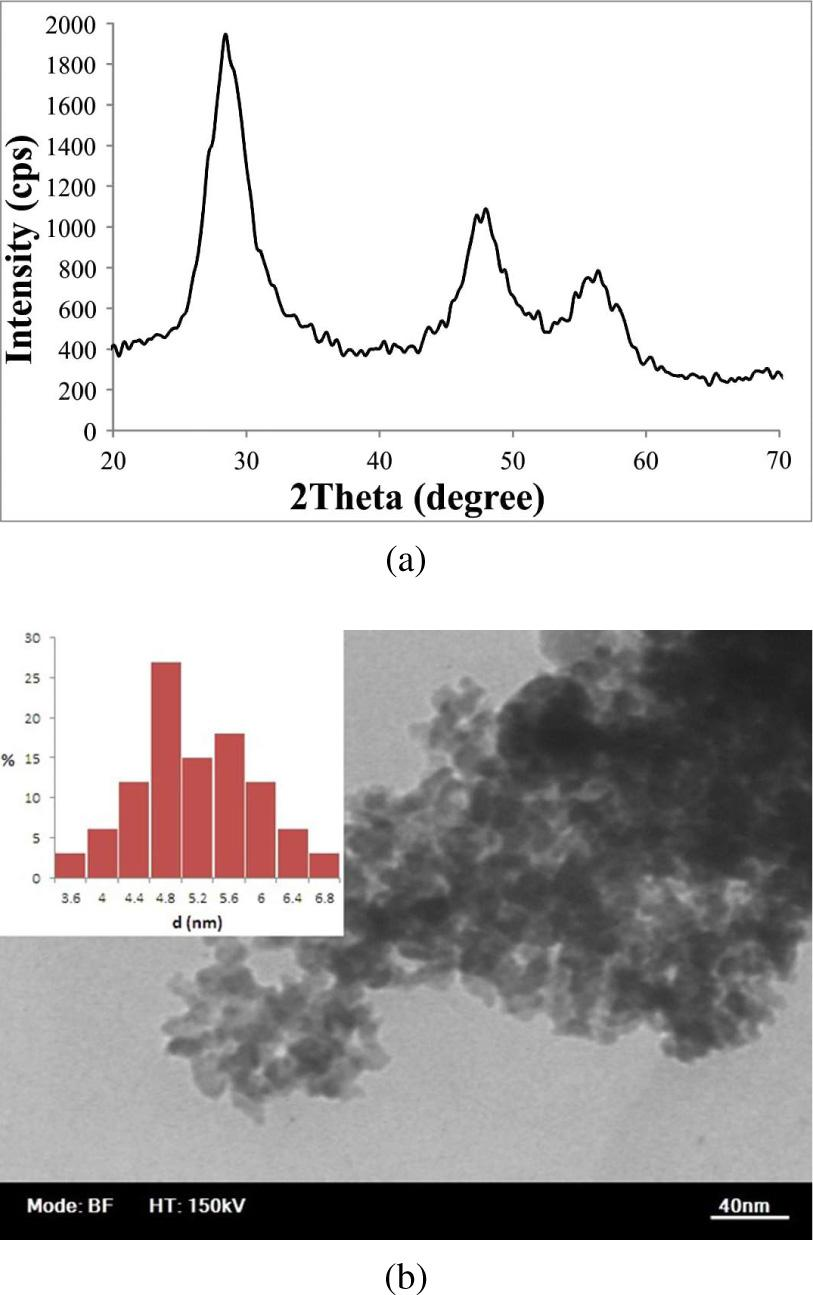(a) XRD pattern and (b) TEM image of sample S.

where D is the calculated crystallite size, k is the dimensionless shape factor with a typical value of 0.9, λ is the X-ray wavelength, β is the full width at half maximum (FWHM) of the considered peak and θ is the Bragg angle at the highest intensity. Considering 2θ = 28.48°, β = 1.67° = 0.029 rad and λ = 1.5408 AA, the estimated mean crystallite size was determined equal to 4.9 nm. This was close to the TEM result of 5.1 nm, as shown in Fig. 1b. Size of the particles can also be calculated from Brus equation  according to the UV-Vis spectrum of the sample S (Fig. 2). The onset of the absorption peak is located at 312 nm which shows a reliable blue shift with respect to the bulk ZnS (340 nm). The Brus equation can be written as :

$E=Eg+h28r2[1me*+1mh*]−1.8e24πeεε0 −0.124e4h2(4πε0εα)2+(1me*+1mh*)$\eqalign{ & E = {E_g} + {{{h^2}} \over {8{r^2}}}\left[ {{1 \over {m_e^*}} + {1 \over {m_h^*}}} \right] - {{1.8{e^2}} \over {4\pi e\varepsilon {\varepsilon _0}}} \cr & {\rm{ }} - {{0.124{e^4}} \over {{h^2}{{\left( {4\pi {\varepsilon _0}{\varepsilon _\alpha }} \right)}^2} + \left( {{1 \over {m_e^*}} + {1 \over {m_h^*}}} \right)}} \cr}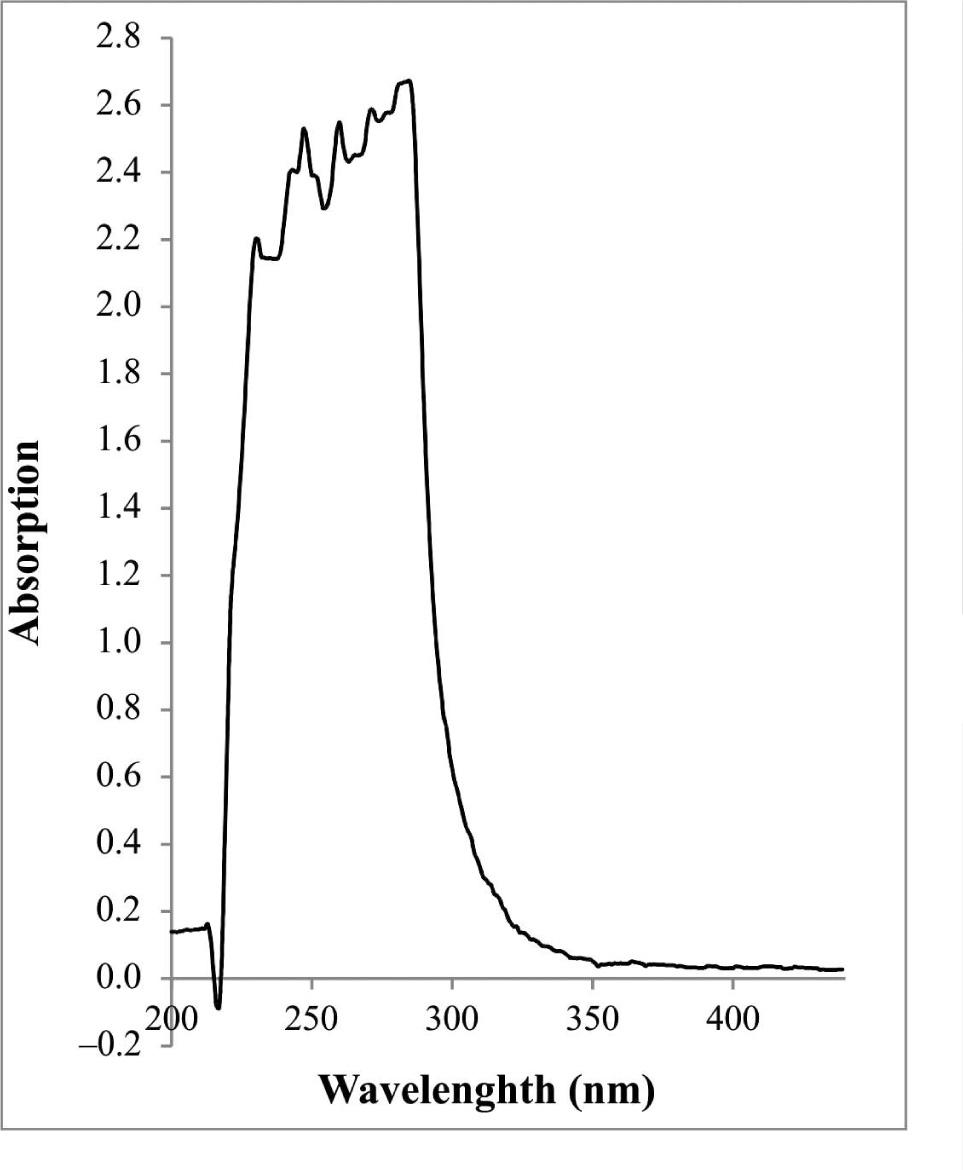UV-Vis spectrum of sample S.

where E and Eg are the onset of the absorption peak of the sample S and the band gap of the bulk material respectively, r is the particle radius, $me*me*$${\rm{m}}_{\rm{e}}^*{\rm{m}}_{\rm{e}}^*$ and $mh*$${\rm{m}}_{\rm{h}}^*$ are the reduced masses of the conduction band electron and valence band hole of bulk ZnS, respectively, ∊0 is the vacuum permittivity and ∊α is the high-frequency dielectric constant of ZnS. These values for ZnS are as follows:

$me*=0.25memh*=0.59mee=1.602×10−19Ch=6.62×10−34J⋅Sε0=8.85418×10−12C2N−1m−2ε=8.3 × 10−12C2N−1m−2Eg=3.68ev=5.89×10−19J$$$\matrix{ {m_e^*} \hfill & = \hfill & {{\rm{0}}{\rm{.25}}{m_e}} \hfill \cr {m_h^*} \hfill & = \hfill & {0.59{m_e}} \hfill \cr e \hfill & = \hfill & {1.602 \times {{10}^{ - 19}}{\rm{C}}} \hfill \cr h \hfill & = \hfill & {6.62 \times {{10}^{ - 34}}{\rm{J}} \cdot {\rm{S}}} \hfill \cr {{\varepsilon _0}} \hfill & = \hfill & {8.85418 \times {{10}^{ - 12}}{{\rm{C}}^2}{{\rm{N}}^{ - 1}}{{\rm{m}}^{ - 2}}} \hfill \cr \varepsilon \hfill & = \hfill & {8.3{\rm{ }} \times {\rm{ 1}}{{\rm{0}}^{ - 12}}{{\rm{C}}^2}{{\rm{N}}^{ - 1}}{{\rm{m}}^{ - 2}}} \hfill \cr {{E_g}} \hfill & = \hfill & {3.68ev = 5.89 \times {{10}^{ - 19}}{\rm{J}}} \hfill \cr }$$

Inserting the data in equation 2, we can obtain the particle radius equal to 2.3 nm. The diameter of the particles is 4.6 nm which is in good agreement with the XRD result, unlike in some other reports that imply the difference between XRD and UV sizes for very small particles .

PL spectra of the samples synthesized at 70 °C and 90 °C are presented in the Fig. 3a and b for the reaction times between 30 and 240 minutes. As it is evident from these figures, the PL intensity increases with the reaction time at all temperatures. As described before, the PL intensity enhancement is mainly due to two factors: increasing particle crystallinity and oxygen adsorption on the activated surface defect sites. As the reaction has been conducted under the air atmosphere, adsorption of oxygen occurred and its effect was therefore dominant . So, the PL intensity enhancement can be considered as a sign of increasing oxygen adsorption. Slight red shifts are observed in the PL spectra of the samples A1 to A6 synthesized at 70 °C which is related to the slight particles growth in the reaction time intervals between 30 and 240 minutes. Such behavior was also observed in the samples synthesized at 90 °C (set B, Table 1).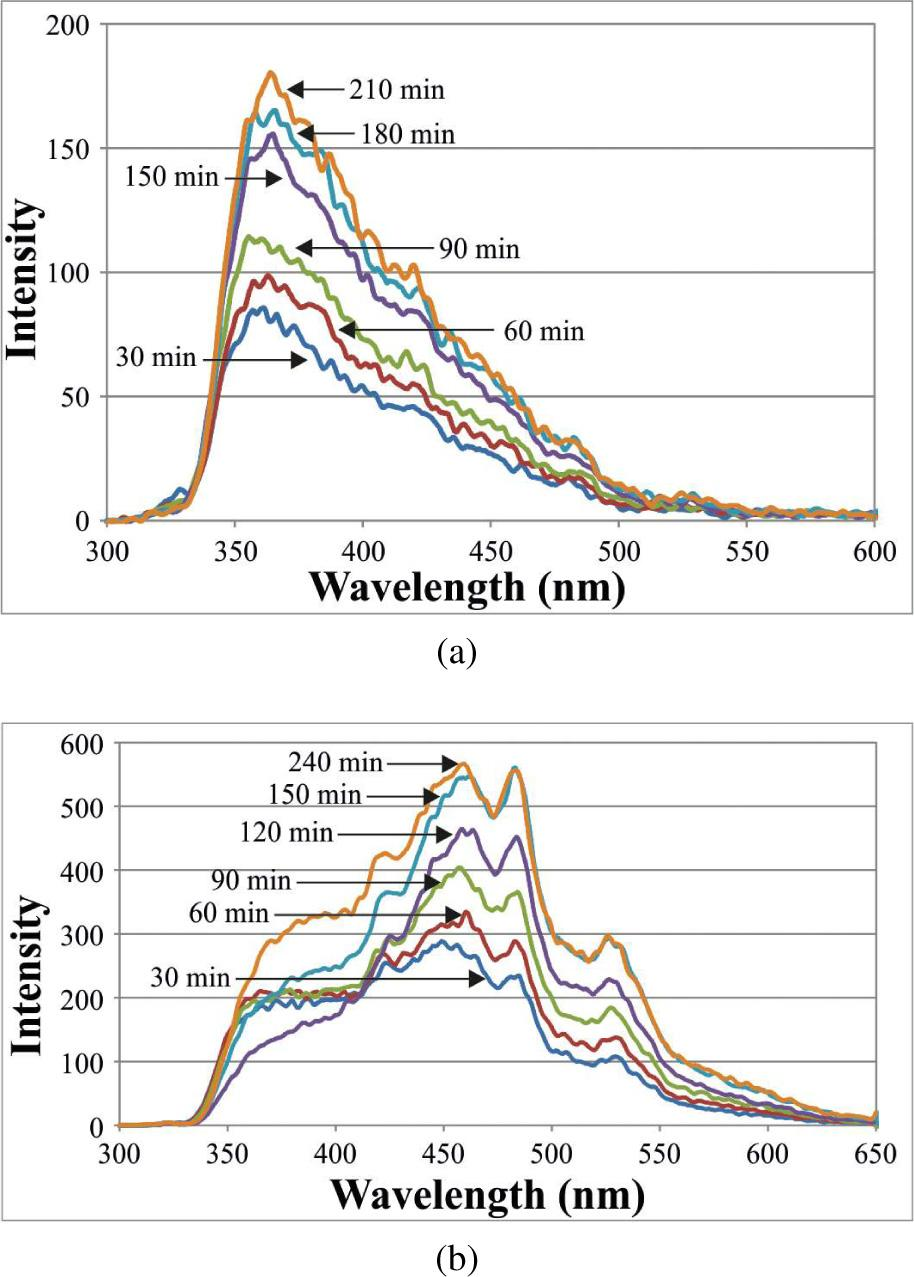PL patterns of samples synthesized at (a) 70 °C and (b) 90 °C. The excitation wavelength is 320 nm.

Fig. 4 shows the diagrams of maximum PL intensity versus reaction time at 70 °C and 90 °C. The PL intensity at 90 °C is higher than at 70 °C for all the reaction times. It is inferred that the oxygen adsorption is the principal reason for the PL intensity enhancement with reaction time.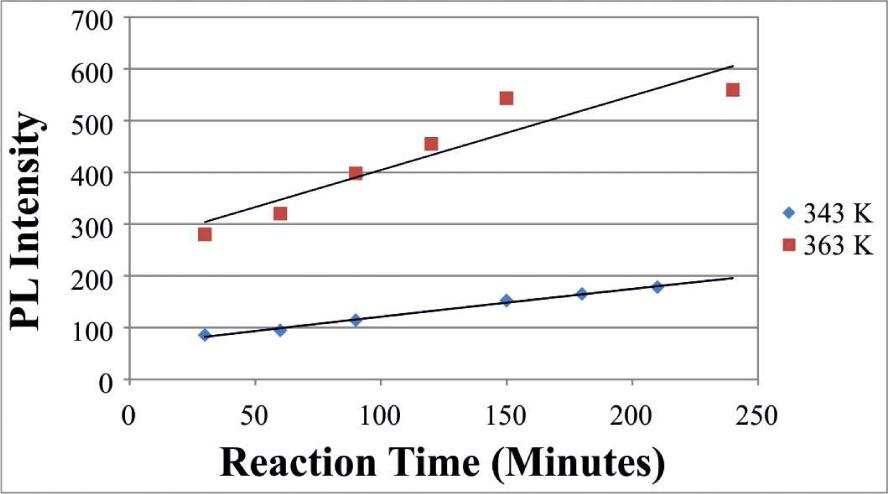The PL intensity of the samples synthesized at 70 °C and 90 °C versus the reaction time.

According to the Chapman-Enskog theory, the diffusion coefficient of gas molecules in the surface adsorption phenomena is proportional to Tn. T is the temperature in Kelvin and n is a number between 1.5 and 1.8, so the diffusion coefficient of the surface oxygen increases with temperature and the oxygen adsorption at 90 °C is higher than at 70 °C. Since the enhancement of the PL intensity is related to increasing of the surface oxygen, assuming that 99 % of the adsorption has been completed until the final reaction time tf, the reaction progression at time t, f(t), can be calculated accordingly:

$f(t)=Imax,tImax,f×100$$$f\left( t \right) = {{{I_{max, t}}} \over {{I_{max, f}}}} \times 100$$

where the f(t) is the fraction of oxygen adsorbed at time t and Imax,t are the maximum PL intensities at time t and at the end of the reaction, respectively. Using this approach, the diagrams f(t) vs. ln(t) and ln[−ln(1 − f(t))] vs. ln(t) have been plotted at two temperatures: 70 °C and 90 °C (Fig. 5 and Fig. 6, respectively). As discussed in our previous works [25, 26], the linearity of the later diagram is the sign of its good coincidence with the Avrami kinetics equation:

$f(t)=1−exp(−ktn)$$$f\left( t \right) = 1 - \exp \left( { - k{t^n}} \right)$$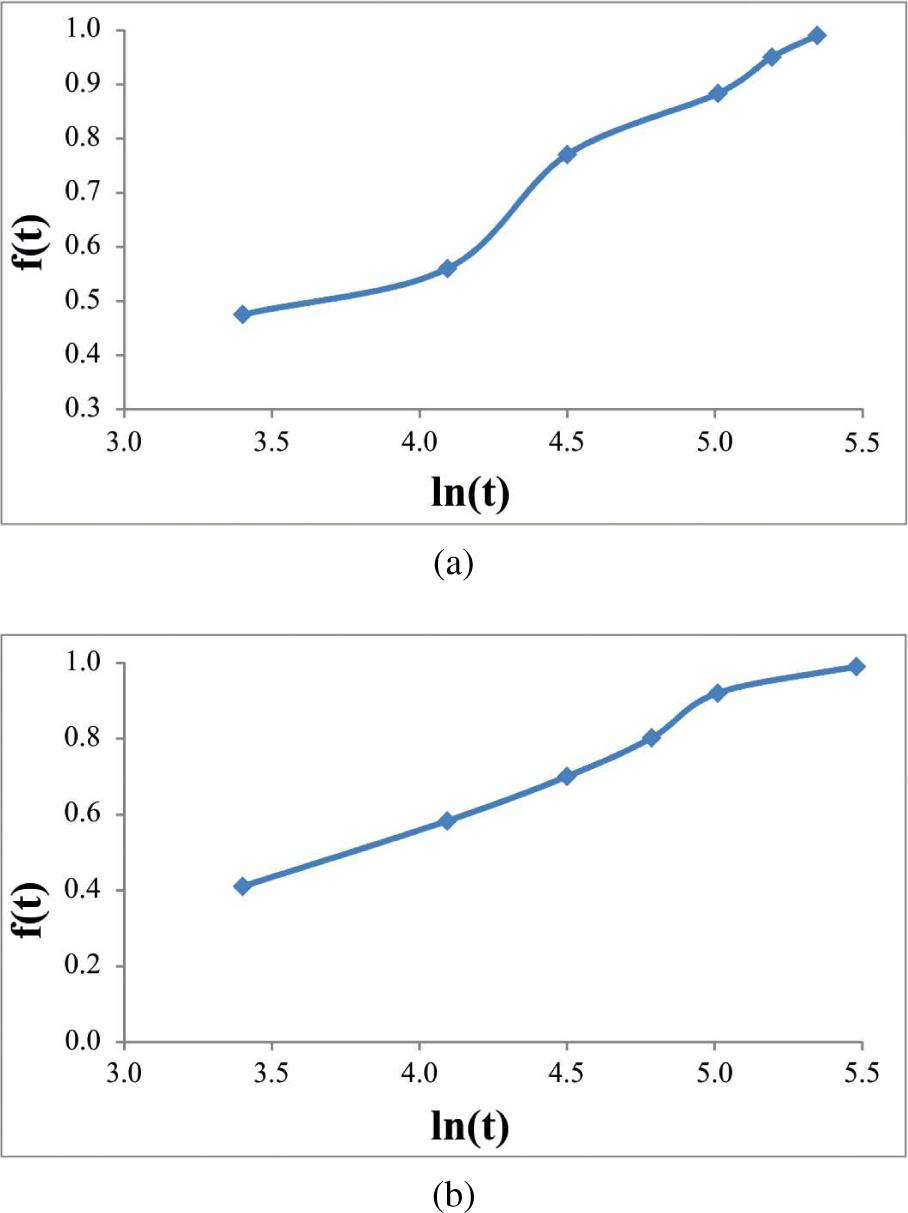The f(t)−ln(t) diagrams for the oxygen absorption process at (a) 70 °C and (b) 90 °C.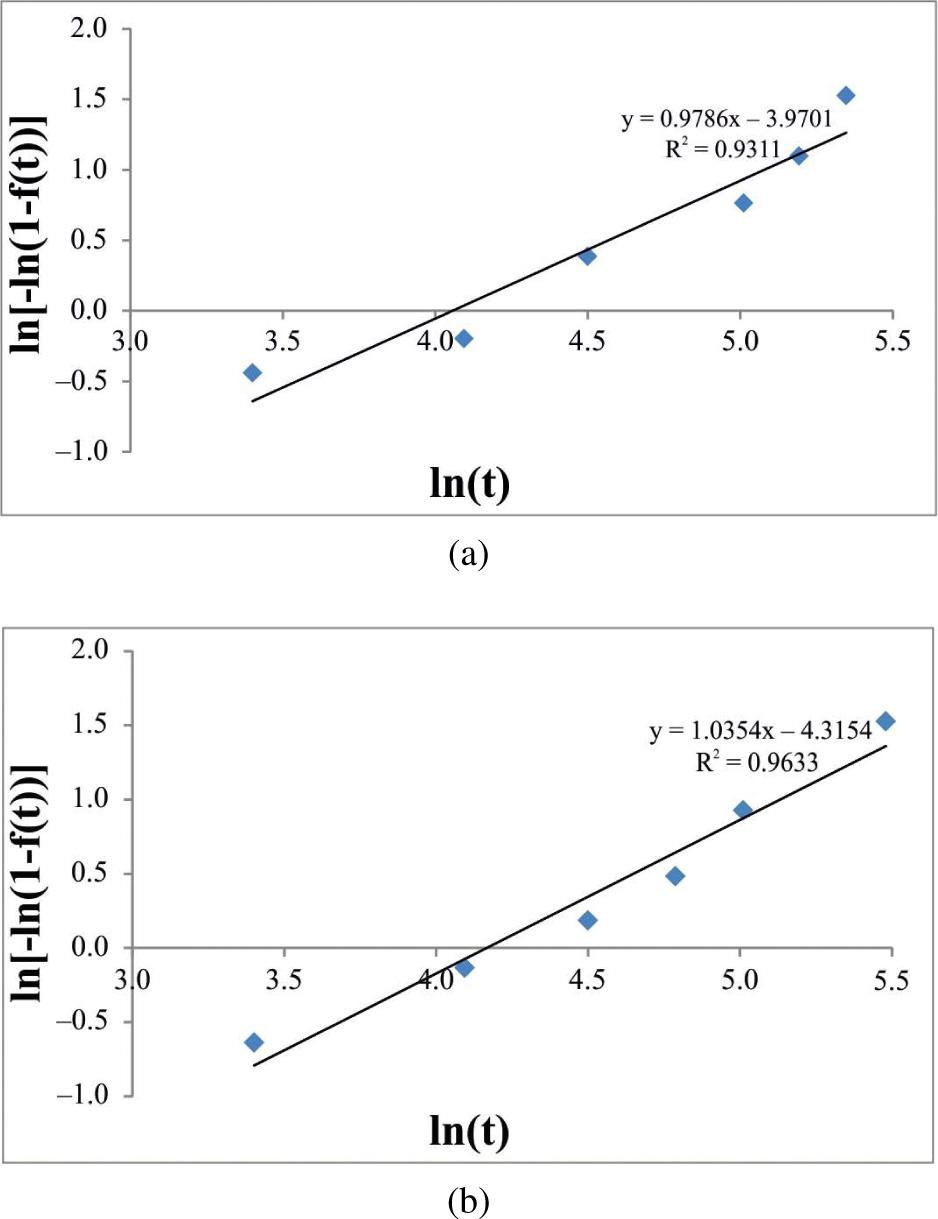The ln[–ln(1 – f(t))]– ln(t) diagrams for the oxygen adsorption process at (a) 70 °C and (b) 90 °C.

where k and n are the kinetics coefficients depending on the experimental conditions, such as temperature, pH, precursor concentrations, etc. As seen in Fig. 6, the obtained plots are semi-linear in shape with the R2 values of 0.9311 and 0.9633 at 70 °C and 90 °C, respectively. Also, the f(t) − ln(t) plots show an “S” type shape which is characteristic of the Avrami transformations with the highest rates at the middle time intervals and a low reaction rate at the beginning and the end of the reaction. So, the kinetics of surface oxygen absorption on the ZnS nanoparticles surface can be stated through the Avrami model. Finally, considering Fig. 6 the kinetics coefficients k and n can be calculated from the trend line slope and intercept with the vertical axis, respectively. So, the related kinetics equations can be written accordingly:

$f343(t)=1−exp(−0.0188t0.9786)$$${f_{343}}\left( t \right) = 1 - \exp \left( { - 0.0188{t^{0.9786}}} \right)$$$f363(t)=1−exp(−0.0133t1.0354)$$${f_{363}}\left( t \right) = 1 - \exp \left( { - 0.0133{t^{1.0354}}} \right)$$

As the PL intensity is strongly affected by the amount of surface adsorbed oxygen, these equations can be useful for achieving a desired PL intensity. This approach, which has been introduced for the first time in this work, can be employed for other semiconductors too.

Conclusions

In the present study, ZnS nanoparticles with the mean particle size of about 5 nm were synthesized via precipitation process. Effects of reaction time and temperature on the PL intensity of the nanoparticles were investigated. Adsorption of oxygen on the samples was suggested to be the main mechanism responsible for the enhancement of the PL intensity. Avrami model was proposed for explanation of the oxygen adsorption on the particles at 70 °C and 90 °C. Relevant kinetics equations were obtained by using some simplifying assumptions. To the best of our knowledge, this is the first time that kinetics of oxygen adsorption on ZnS nanoparticles has been studied as the main mechanism responsible for the PL intensity enhancement.

#### Experimental conditions of synthesizing samples A1 to A6 and B1 to B6.

t [min] →306090120150180210240
T [°C] ↓
70A1A2A3(S)A4A5A6
90B1B2B3B4B5B6

Li J., Xu Y., Liu Y., Wu D., Sun Y., China Particuology, 2 (2004), 266.LiJ.XuY.LiuY.WuD.SunY.China Particuology2200426610.1016/S1672-2515(07)60072-4Search in Google Scholar

Dahbi N., Arafah D.E., Energ. Procedia, 18 (2012), 85.DahbiN.ArafahD.E.Energ. Procedia1820128510.1016/j.egypro.2012.05.020Search in Google Scholar

Miao S., Liu S., Han B., Yang H., Miao Z., Sun Z., J. Colloid. Interf. Sci., 301 (2006), 116.MiaoS.LiuS.HanB.YangH.MiaoZ.SunZ.J. Colloid. Interf. Sci301200611610.1016/j.jcis.2006.04.080Search in Google Scholar

Dong F., Guo Y., Zhang J., Li Y., Yang L., Fang Q., Fang H., Jiang K., Mater. Lett., 97 (2013), 59.DongF.GuoY.ZhangJ.LiY.YangL.FangQ.FangH.JiangK.Mater. Lett9720135910.1016/j.matlet.2013.01.029Search in Google Scholar

Li L., Bian R., Ding Y., Yu M., Yu D., Mater. Chem. Phys., 113 (2009), 905.LiL.BianR.DingY.YuM.YuD.Mater. Chem. Phys113200990510.1016/j.matchemphys.2008.08.050Search in Google Scholar

Dimitrova V., Tale J., Thin Solid Films, 365 (2000), 134.DimitrovaV.TaleJ.Thin Solid Films365200013410.1016/S0040-6090(99)01089-5Search in Google Scholar

Pathak C.S., Mishra D.D., Agarwala V., Mandal M.K., Mat. Sci. Semicon. Proc., 16 (2013), 525.PathakC.S.MishraD.D.AgarwalaV.MandalM.K.Mat. Sci. Semicon. Proc16201352510.1016/j.mssp.2012.10.005Search in Google Scholar

She Y.Y., Yang J., Qiu K., T. Nonferr. Metal. Soc., 20 (2010) 211.SheY.Y.YangJ.QiuK.T. Nonferr. Metal. Soc202010211Search in Google Scholar

Uekawa N., Matsumoto T., Kojima T., Shiba F., Kakegawa K., Colloid. Surface. A, 361 (2010), 132.UekawaN.MatsumotoT.KojimaT.ShibaF.KakegawaK.Colloid. Surface. A361201013210.1016/j.colsurfa.2010.03.014Search in Google Scholar

Su J., Gao F., Hou L., Mater. Lett., 92 (2013), 206.SuJ.GaoF.HouL.Mater. Lett92201320610.1016/j.matlet.2012.11.011Search in Google Scholar

Seoudi R., Shabaka A., Eisa W.H., Anies B., Farage N.M., Physica B, 405 (2010), 919.SeoudiR.ShabakaA.EisaW.H.AniesB.FarageN.M.Physica B405201091910.1016/j.physb.2009.10.015Search in Google Scholar

Charinpanitkul T., Chanagul A., Dutta J., Rungsardthong U., Tanthapanikakoon W., Sci. Technol. Adv. Mat., 6 (2005), 266.CharinpanitkulT.ChanagulA.DuttaJ.RungsardthongU.TanthapanikakoonW.Sci. Technol. Adv. Mat6200526610.1016/j.stam.2005.02.005Search in Google Scholar

Li Y., Chen J., Zhu C., Wang L., Zhao D., Zhuo S., Wu Y., Spectrochim. Acta A, 60 (2004), 1719.LiY.ChenJ.ZhuC.WangL.ZhaoD.ZhuoS.WuY.Spectrochim. Acta A602004171910.1016/j.saa.2003.09.01215248943Search in Google Scholar

Dutta K., Manna S., De S.K., Synthetic. Met., 159 (2009), 315.DuttaK.MannaS.DeS.K.Synthetic. Met159200931510.1016/j.synthmet.2008.09.003Search in Google Scholar

Cowles C.L., Zhu X., Biosens. Bioelectron., 30 (2011), 342.CowlesC.L.ZhuX.Biosens. Bioelectron30201134210.1016/j.bios.2011.09.03422014622Search in Google Scholar

Loukanov A.R., Dushkin C.D., Papazova K.I., Kirov A.V., Abrashev M.V., Adachi E., Colloid. Surface. A, 245 (2004), 9.LoukanovA.R.DushkinC.D.PapazovaK.I.KirovA.V.AbrashevM.V.AdachiE.Colloid. Surface. A2452004910.1016/j.colsurfa.2004.06.016Search in Google Scholar

Geszke M., Murias M., Medjahdi G., Korczyncki J., Mortiz M., Lulek J., Schneider R., Acta Biomater., 7 (2011), 1327.GeszkeM.MuriasM.MedjahdiG.KorczynckiJ.MortizM.LulekJ.SchneiderR.Acta Biomater72011132710.1016/j.actbio.2010.10.01220965282Search in Google Scholar

Gaceur M., Giraud M., Hemadi M., Nowak S., Menguy N., Quisefit J.P., David K., Jahanbin T., Benderbous S., BOISSI ˇCre M., Ammar S., J. Nanopart. Res., 14 (2012), 932.GaceurM.GiraudM.HemadiM.NowakS.MenguyN.QuisefitJ.P.DavidK.JahanbinT.BenderbousS.Boissi ˇCreM.AmmarS.J. Nanopart. Res14201293210.1007/s11051-012-0932-3Search in Google Scholar

Wang S., Jarret B.R., Kauzlarich S.M., Lourie A.Y., J. Am. Chem. Soc., 129 (2007), 3848.WangS.JarretB.R.KauzlarichS.M.LourieA.Y.J. Am. Chem. Soc1292007384810.1021/ja065996d253369317358058Search in Google Scholar

Angelo P.D., Keonfli R., Farnood R.R., J. Lumin., 136 (2013), 100.AngeloP.D.KeonfliR.FarnoodR.R.J. Lumin136201310010.1016/j.jlumin.2012.10.043Search in Google Scholar

Murugadoss G., Rajamannan B., Ramasamay V., J. Lumin., 130 (2010), 2032.MurugadossG.RajamannanB.RamasamayV.J. Lumin1302010203210.1016/j.jlumin.2010.05.022Search in Google Scholar

Zhuang J., Zhang X., Wang G., Li D., Yang W., Li T., J. Mater. Chem., 13 (2003), 1853.ZhuangJ.ZhangX.WangG.LiD.YangW.LiT.J. Mater. Chem132003185310.1039/b303287fSearch in Google Scholar

Yu J.H., KWon S.H., Petrasek Z., Park O.K., Jun S.W., Shin K., Nat. Mater., 12 (2013), 359.YuJ.H.KwonS.H.PetrasekZ.ParkO.K.JunS.W.ShinK.Nat. Mater12201335910.1038/nmat356523416726Search in Google Scholar

Liu B.R., Winiarz J.G., Moon J.S., LO S.Y., huang Y. W., Lee H.J., Colloid. Suface. B, 111 (2013), 162.LiuB.R.WiniarzJ.G.MoonJ.S.LoS.Y.HuangY. W.LeeH.J.Colloid. Suface. B111201316210.1016/j.colsurfb.2013.05.03823792556Search in Google Scholar

Ahmadi R., Masoudi A., Madaah Hosseini H.R., Gu N., Ceram. Int., 39 (2013), 4999.AhmadiR.MasoudiA.Madaah HosseiniH.R.GuN.Ceram. Int392013499910.1016/j.ceramint.2012.11.097Search in Google Scholar

Ahmadi R., Madaah Hosseini H.R., Masoudi A., J. Min. Metall. B., 47 (2011), 211.AhmadiR.Madaah HosseiniH.R.MasoudiA.J. Min. Metall. B47201121110.2298/JMMB110330010ASearch in Google Scholar

• #### Growth, spectral, optical, dielectric and third order nonlinear studies on 2-amino 4-methylpyridinium salicylate single crystals

Recommended articles from Trend MD